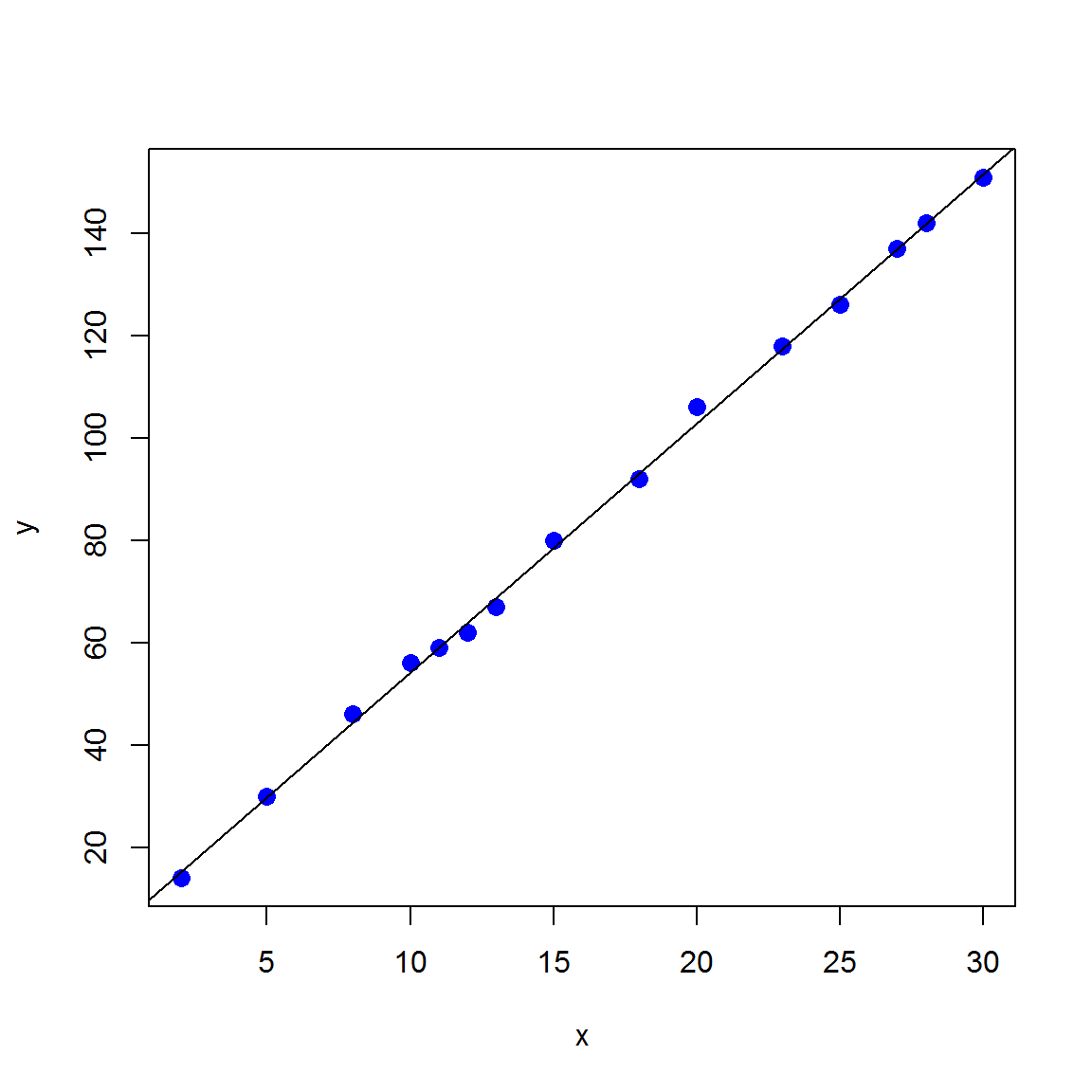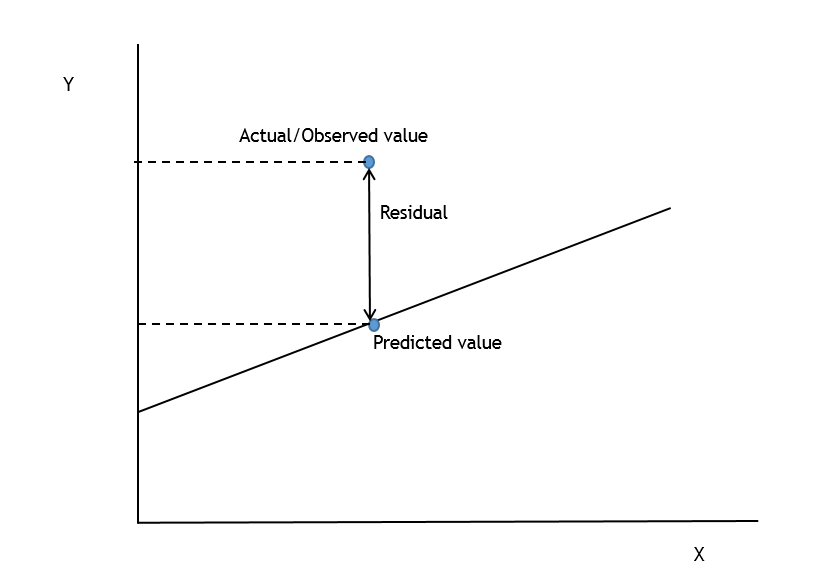# Overview

The objective of this tutorial is to provide students with an introduction to linear regression using R. This tutorial covers the following topics:

• General overview of simple and multiple linear regression
• Running simple and multiple linear regression using R
• Testing the significance of variables
• Measures of model fit
• Residual Analysis
• Identifying outliers, leverage, and influential points

# What is Simple Linear Regression?

Consider the following data pairs which are in the form $$(x_1,y_1 ),(x_2,y_2), \ldots ,(x_n,y_n)$$ for two variables $$x$$ and $$y$$.

\begin{aligned} (2,14), (5,30), (8,46), (10, 56), (11,59), (12,62), (13,67),(15,80),& \\ (18,92), (20,106), (23,118), (25,126), (27,137), (28,142), (30,151)& \end{aligned}

The scatter plot is shown belowThe goal of simple linear regression is to develop a linear function to explain the variation in $$y$$ based on the variation in $$x$$. For the above data, the following linear function best explains the relationship between $$y$$ and $$x$$

$y = 5.54 + 4.87x$

In this model 5.54 is called the intercept and 4.87 is called the slope. How did we arrive at the values of the slope and the intercept. How do we know $$y = 5.54 + 4.87x$$ is the best model? The next section attempts to answer these questions.

# Ordinary Least Squares Regression Line

This section is inspired by the example in Chapter 4 of Barreto and Howland (2006). Go to the folowing website ShinyApp. Change the values of intercept and slope. Visually try to find the intercept and slope which best represents the data.

Let us say the best value we got was 14 for the intercept and 12 for the slope.

$y = 14 + 12x$

Let us look at $$x=5$$. Corresponding to $$x=5$$ there are two values of $$y$$, the actual observed value and the predicted value. The predicted value of $$y$$ is $$14 + 12 \times 5 = 74$$ and the actual value of $$y$$ is 79. The residual is the difference between the actual and predicted value.Now observe how the Residual Sum of Squares changes with intercept and slope. What is the Residual Sum of Squares?

For a specific value of slope and intercept:

• For each value of $$x$$, calculate the residual which is the difference between the observed and the predicted value.
• Square the residuals and sum them up across all values of $$x$$. This can be thought of as a measure of error.

Why do we square the residuals? This is to prevent negative residuals and positive residuals from canceling each other out. Observations which are far off from the line are poorly predicted. It does not matter if they are above or below the line.

The goal in linear regression is to choose the slope and intercept such that the Residual Sum of Squares is as small as possible. Excel and R have functions which will automatically calculate the values of the slope and the intercept which minimizes the Residual Sum of Squares.

If the shiny app is not working, you can repeat the above exercise using the excel workbook Reg.xls. Go to the sheet ByEye and minSSRes.

# Mathematical Foundations of Linear Regression

In simple linear regression we are fitting a function of the form:

$y = \beta_0 + \beta_1 x + \epsilon$

$$\epsilon$$ corresponds to the error term which is assumed to have zero mean, constant variance, uncorrelated, and normally distributed. If the above assumptions are not valid, then the regression model is unacceptable.

In a multiple linear regression we are fitting a function of the form:

$y = \beta_0 + \beta_1 x_1 + \beta_2 x_2 + \ldots + \beta_n x_n + \epsilon$

$$y$$ is called the dependent variable.

$$x_1,x_2, \ldots ,x_n$$ are called independent variables. They are also referred to as predictors, covariates, and regressors in statistical literature.

$$\beta_0,\beta_1,\beta_2, \ldots , \beta_n$$ are called regression coefficients.

Note that because of the assumptions on $$\epsilon$$, $$y$$ will have a normal distribution with expected value:

$E[y \mid x] = \beta_0 + \beta_1 x_1 + \beta_2 x_2 + \ldots + \beta_n x_n$ If the constant variance of the error term is $$\sigma^2$$, then the variance of $$y$$ is:

$Var[y \mid x] = \sigma^2$

# Simple Linear Regression in R

Open the dataset WatCon.csv in RStudio. This dataset corresponds to example 6.12 in Tang and Ang (2007). We want to study water consumption as a function of population.

> setwd("C:/Users/avinash/Dropbox/Teaching/Tutorial/Linear Regression")
> WCData <- read.csv("WatCon.csv", header = TRUE)
> WCData
   CITY    POP  WC
1     1  50000 100
2     2 100000 110
3     3 200000 110
4     4 250000 113
5     5 300000 125
6     6 400000 130
7     7 500000 130
8     8 600000 145
9     9 700000 155
10   10 800000 150

The first key assumption in linear regression is the existence of a linear relationship between $$y$$ and $$x$$. To verify this, make sure the scatter plots looks linear.

> plot(WCData$POP, WCData$WC, xlab = "Population", ylab = "Water Consumption", pch = 16, cex = 1.3, col = "blue")